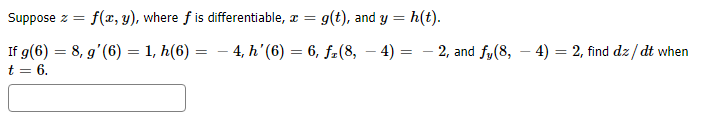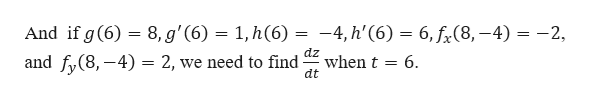# h(t)f(x, y), where f is differentiable, ax =g(t), and ySuppose z- 4, h' (6) 6, f2(8,If g(6) 8, g'(6) = 1, h(6)t 64)- 2, and fy(8, - 4) 2, find dz/dt when

Question
1 viewshelp_outlineImage Transcriptioncloseh(t) f(x, y), where f is differentiable, ax = g(t), and y Suppose z - 4, h' (6) 6, f2(8, If g(6) 8, g'(6) = 1, h(6) t 6 4) - 2, and fy(8, - 4) 2, find dz/dt when fullscreen
check_circle

Step 1

We are given that z = f(x, y), where f is di...help_outlineImage TranscriptioncloseAnd if g (6) 8, g'(6) = 1, h(6) = -4, h'(6) = 6, fx(8,-4) = -2, dz when t 6 dt and fy(8,-4) = 2, we need to find _ fullscreen

### Want to see the full answer?

See Solution

#### Want to see this answer and more?

Solutions are written by subject experts who are available 24/7. Questions are typically answered within 1 hour.*

See Solution
*Response times may vary by subject and question.
Tagged in
MathCalculus

### Functions